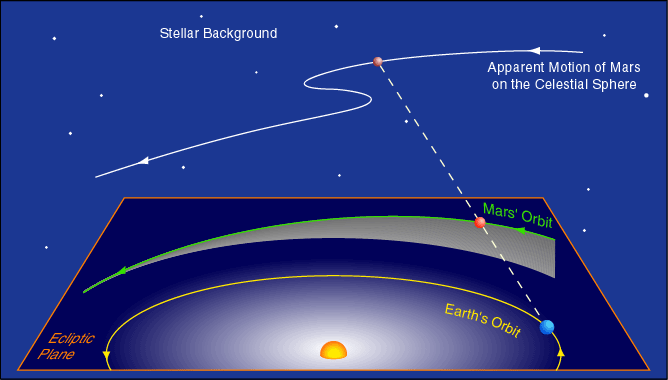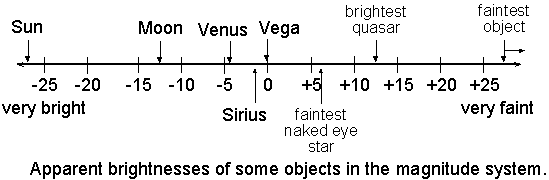# Basics of Astronomy

Astronomy: - Astronomy is the science of measuring the positions and characteristics of heavenly bodies or you can say it is mainly depends on observation
Astrophysics: - Astophysics mainly consists of theorotical part or you can say astrophysics is the application of physics to understand astronomy.
Cosmogony: - It is the branch of science that deals with the origin of the universe, especially the solar system.

## Units Used in Astronomy

### 1. Distance

a) Astronomical Unit (AU)
The distance between earth and sun is taken as 1 AU. It is convenient to express distance between planets and sun in AU
$1\phantom{\rule{thinmathspace}{0ex}}AU=1.496×{10}^{11}\phantom{\rule{thinmathspace}{0ex}}m$$1 \,AU=1.496\times10^{11}\, m$

a) Lightyear (ly)
The distance travelled by light in one year is called 1 ly.
$1\phantom{\rule{thinmathspace}{0ex}}ly=9.461×{10}^{15}\phantom{\rule{thinmathspace}{0ex}}m$$1 \,ly=9.461\times10^{15}\, m$

a) Parsec (pc)
It is the distance at which an arc 1 AU subtends an angle of 1”. It is convenient to express distance of stars from earth by parallax metod.
$1\phantom{\rule{thinmathspace}{0ex}}pc=3.086×{10}^{16}\phantom{\rule{thinmathspace}{0ex}}m$$1\,pc=3.086\times10^{16}\, m$ [distance\,in\,parsecs =\frac{1}{\theta(in “) made\,to\,earth’s\,orbital\,radius}]

### 2. Mass

For planets we use jupiter’s mass which is equal to $1.899×{10}^{27}\phantom{\rule{thinmathspace}{0ex}}kg$$1.899\times10^{27}\,kg$. Mass of stars is expressed in Solar mass which is equal to $1.98×{10}^{30}\phantom{\rule{thinmathspace}{0ex}}kg$$1.98\times10^{30}\,kg$

### 3. Constants

a) $G=6.673×{10}^{-11}\phantom{\rule{thinmathspace}{0ex}}N{m}^{2}k{g}^{-2}$$G=6.673\times10^{-11} \,Nm^{2}kg^{-2}$
b) $c=299,792,458\phantom{\rule{thinmathspace}{0ex}}m{s}^{-1}\approx 3×{10}^{8}\phantom{\rule{thinmathspace}{0ex}}m{s}^{-1}$$c=299,792,458\,ms^{-1}\approx3\times10^8\,ms^{-1}$
c) Solar Luminosity$\phantom{\rule{thinmathspace}{0ex}}=3.828×{10}^{26}\phantom{\rule{thinmathspace}{0ex}}W$$\,=3.828 \times 10^{26} \,W$
d) Solar Constant $=1370\phantom{\rule{thinmathspace}{0ex}}W{m}^{-2}$$=1370\,Wm^{-2}$

## Observing Planet

### Transit

Planet moving over solar disk. It happens only for Mercury and Venus

### Occulation

Moon/Planets Eclipsing distant object is known as occulation

Planet appears moving in opposite direction

### Other Terms

Inferior Planet - Has an orbit that is closer than the Earth-Sun distance. (Mercury and Venus)

Superior Planet - Has an orbit that is farther than the Earth-Sun distance. (Mars, Jupiter, Saturn, Uranus, Neptune)

Opposition - A planetary position in which the Sun, the Earth and a superior planet are aligned in that order and in a straight line. This is also the best time to observe a planet because:
• it is at its closest approach to Earth.
• the planet rises just as the Sun is setting, allowing observation any time during the night.

Quadrature - A planetary position in which the Sun, the Earth and a superior planet form a right angle with the Earth at the vertex.

Conjunction - A planetary position in which the Earth, the Sun and the planet are aligned in that order and in a straight line.
For an inferior planet this is called a superior conjunction.
When the order is the Earth, the inferior planet and the Sun, it is an inferior conjunction.
A conjunction is the worst time to observe a planet because it is lost in the Sun’s brilliance.

Greatest Elongation - Point at which an inferior planet has the greatest visual distance from the Sun. (Mercury 28º Venus 47º)
During Greatest Eastern Elongation an inferior planet is visible at sunset and is visible before sunrise during Greatest Western Elongation.

Retrograde motion is the apparent motion of a planet in a direction opposite to that of other bodies within its system, as observed from a particular vantage point. Direct motion or prograde motion is motion in the same direction as other bodies.#### Reason for Apparent Retrograde Motion## Quantifying Brightness of stars

### Apparent Magnitude

As it appears from earth [m=-2.5\log{\left(\frac{flux_{object}}{flux_{reference}}\right)}] [m_1-m_2=-2.5\log{\left(\frac{f_1}{f_2}\right)}]
Note: Magnitude Value increases Brightness of star decreases.
Our eyes are limited to a magnitude 6 stars.### List of 50 brightest starsList

### Surface Brightness

It is magnitude of brightness of extended object [s=m+2.5\log{A}][A\rightarrow area\,in\,arcsec^2]
<h3>Absolute Magnitude</h3>
all stars considered at 10pc [M=m+5-5\log{D}][D\rightarrow distance\,between\,star\,and\,earth] for planets distance is considered to be 1 A.U.

## Some other terms

Never setting stars or Circumpolar stars These are those stars which never go below horizon
Never rising stars Theses stars never rise